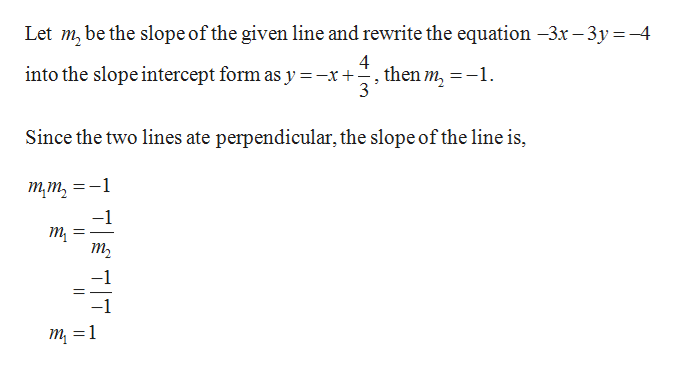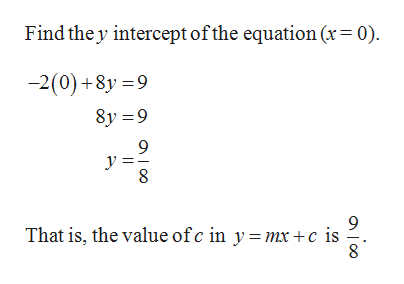Find an equation for the line perpendicular to the line −3x−3y=−4−3x−3y=−4 having the same y-intercept as −2x+8y=9−2x+8y=9.

Question

Find an equation for the line perpendicular to the line −3x−3y=−4−3x−3y=−4 having the same y-intercept as −2x+8y=9−2x+8y=9.

Step 1

It is known that, let m1 and m2 be the slope of the perpendicular lines then m1×m2 = –1.help_outlineImage TranscriptioncloseLet m, be the slope of the given line and rewrite the equation -3x -3y-4 4 into the slope intercept form as y =-x+-, then m, = -1. 3 Since the two lines ate perpendicular, the slope of the line is, тт, _ -1 т т —1 7TT fullscreen
Step 2help_outlineImage TranscriptioncloseFind the y intercept ofthe equation (x= 0) -2(0)+8y 9 8y 9 9 9 That is, the value ofc in y =mx +c is fullscreen

Want to see the full answer?

See Solution

Want to see this answer and more?

Our solutions are written by experts, many with advanced degrees, and available 24/7

See Solution
Tagged in

Other